# Chord

Point on the circle is the end point of diameter and end point of chord length of radius. What angle between chord and diameter?

Correct result:

α =  60 °

#### Solution:

$\alpha =6{0}^{\circ }$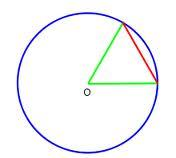We would be pleased if you find an error in the word problem, spelling mistakes, or inaccuracies and send it to us. Thank you!Tips to related online calculators

#### You need to know the following knowledge to solve this word math problem:

We encourage you to watch this tutorial video on this math problem:

## Next similar math problems:

• ClassIn 7.C clss are 10 girls and 20 boys. Yesterday was missing 20% of girls and 50% boys. What percentage of students missing?
• CircleWhat is the radius of the circle whose perimeter is 6 cm?
• 3-bracketMay be the largest angle in the triangle less than 20°?
• DiagonalCan a rhombus have the same length diagonal and side?
• CableCable consists of 8 strands, each strand consists of 12 wires with diameter d = 0.5 mm. Calculate the cross-section of the cable.
• Coal mine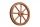The towing wheel has a diameter of 1.7 meters. How many meters does the elevator cage lower when the wheel turns 32 times?
• AnglesIn the triangle ABC, the ratio of angles is: a:b = 4: 5. The angle c is 36°. How big are the angles a, b?
• 3-bracket 3Two angles in a triangle are 90° and 60°. Has triangle at least two equal sides?
• Isosceles - isoscelesIt is given a triangle ABC with sides /AB/ = 3 cm /BC/ = 10 cm, and the angle ABC = 120°. Draw all points X such that true that BCX triangle is an isosceles and triangle ABX is isosceles with the base AB.
• Angles in triangle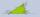Calculate the alpha angle in the triangle if beta is 61 degrees and 98 gamma degrees.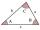The size of two internal angles of a triangle ABC are α=6/18π and β=7/18π. Calculate the size of the third angle.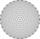What is the sum of interior angles 8-gon? What is the internal angle of a regular convex 8-polygon?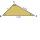How do I find the triangle type if the angle ratio is 2:3:7 ?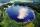Across the circle lakepasses through its center bridge over the lake. At three different locations on the lake shore are three fishermen A, B, C. Which of fishermen see the bridge under the largest angle?The triangles ABC and A'B'C 'are similar. In the ABC triangle, the two angles are 25° and 65°. Explain why in the triangle A'B'C 'is the sum of two angles of 90 degrees.Side of the triangle inscribed in a circle is a chord passing through circle center. What size are the internal angles of a triangle, if one of them is 40°?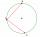There is a given circle k (center S, radius r). From point A which lies on circle k are starting two chords of length r. What angle does chords make? Draw and measure.